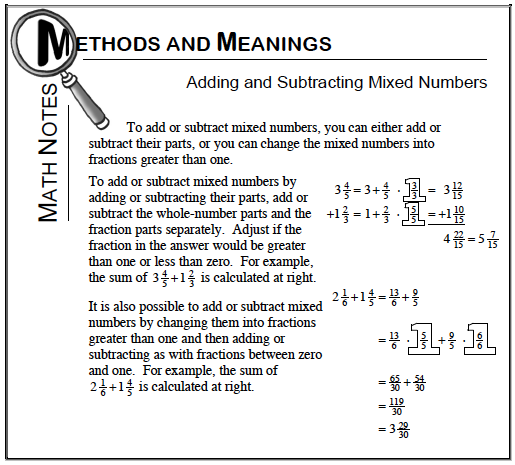### Home > MC1 > Chapter 8 > Lesson 8.2.3 > Problem8-56

8-56.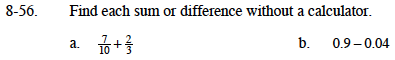For part (a), review the Math Notes box below to find help with adding fractions.

To add the fractions together, we can start by finding the least common multiple of 3 and 10 so we can use that number as a common denominator. This table lists multiples of 3 and 10.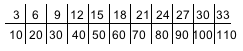LCM is 30. We can rewrite each fraction to have a denominator of 30 by using Giant Ones, as shown below. Can you add the fractions now?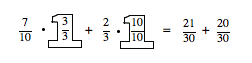$\frac{41}{30}\text{ or }1\frac{11}{30}$

Whenever adding or subtracting decimals, be sure to line up the decimal point! It may help to write this problem like this:

$\:\:\:\:0.90\:$

$\underline{-0.04\:}$

$\:\:\:\:?.??\:$

Also, remember that the decimal point will fall in the same place in your answer.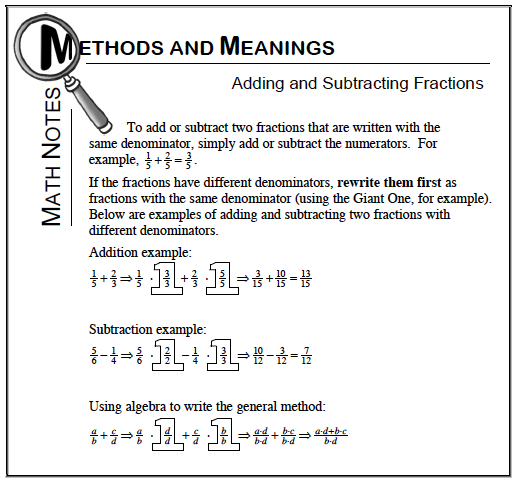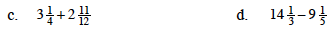Refer to the Math Notes box below.

Refer to the Math Notes box below.